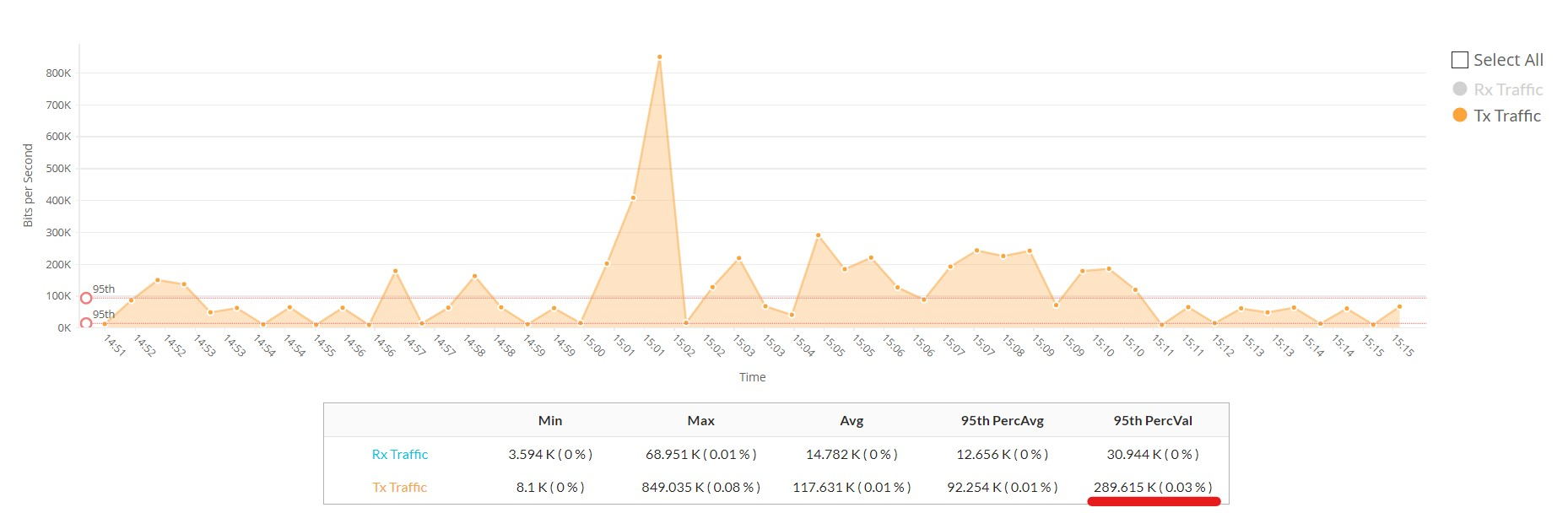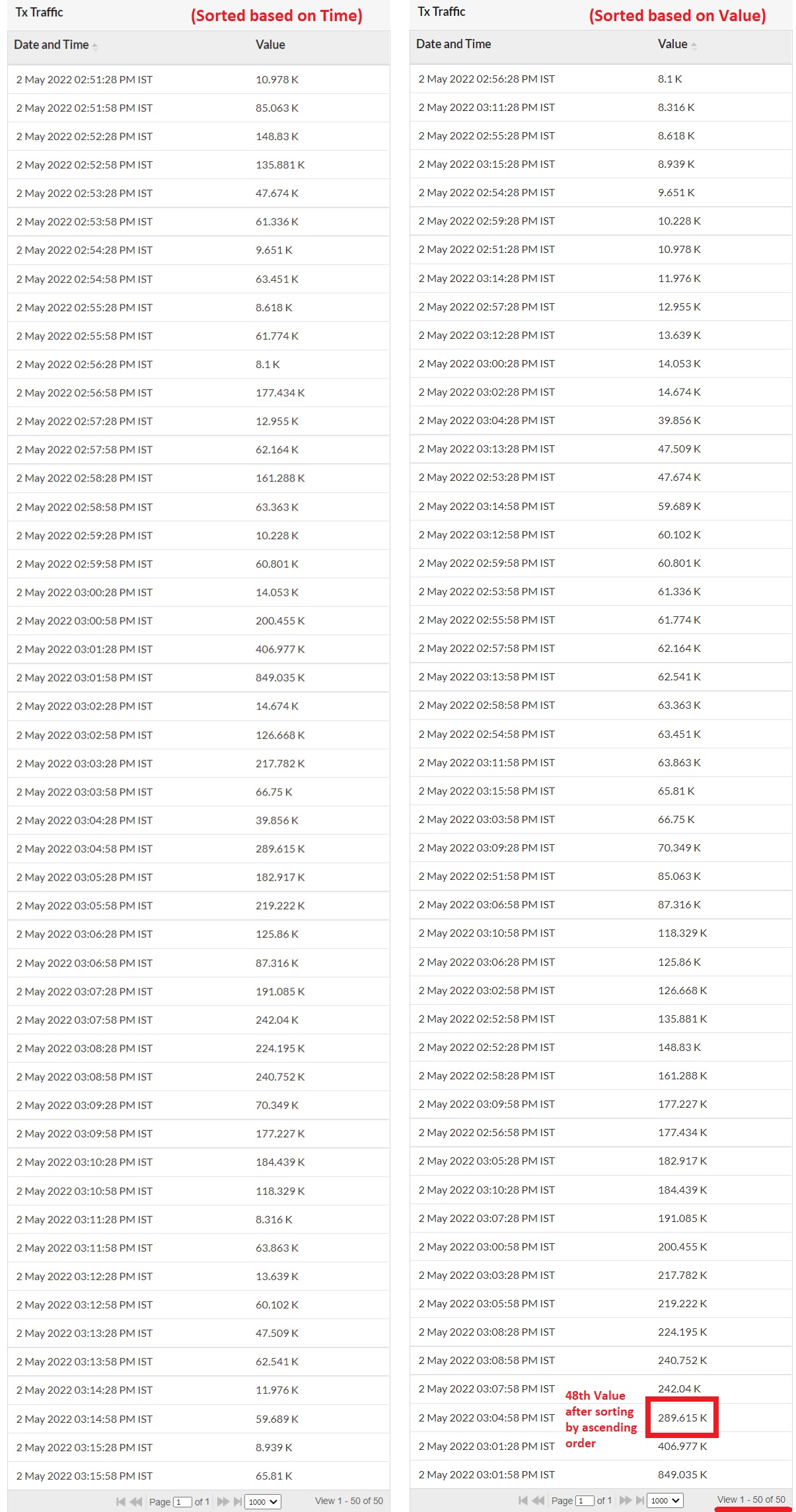• Network Performance
• Server & Virtualization
• Network Visualization
• Network Fault Management
• Data Center Management
• Storage Management
• Reports
• Multi-site & Remote Monitoring
• Mobile Application
• Network Monitoring Basics
• OpManager Alternatives
• Network Access Control

# 95th Percentile Calculation

The 95th percentile is a number that is greater than 95% of the numbers in a given set. The reason this statistic is so useful in measuring data throughput is that it gives a very accurate picture of the maximum traffic generated on an interface. This is a standard measure used in interpreting performance data. This 95th percentile is the highest value left when the top 5% of a numerically sorted set of collected data is discarded. It is used as a measure of the peak value used when one discounts a fair amount for transitory spikes. This makes it markedly different from the average.

To calculate the 95th percentile value,

• Note the number of values in the sample (K).
• Calculate N. N = K x 0.95.
• Sort the samples in ascending order. Now, the Nth value in the sorted list will be the 95th percentile value.
• Also, the average of first N values will be the 95th percentile average.

The following example will help you understand it better. In the report attached below, we have 50 samples taken from Tx traffic graph.

Here,

• The total number of entries (K) in the report is 50. So, K = 50.
• Since the 95th percentile value (N) is being calculated, multiply the number of entries (K) with 0.95.
Therefore, N = 50 x 0.95 = 47.5.
• In the below image, the section marked as 'Sorted based on Value' has the values arranged in ascending order. The 48th value in the sorted list is 289.615K. This is The 95th percentile value.
• Similarly, the 95th percentile average can be calculated by taking the average of the values lesser than 289.615 (the 95th percentile value). ((8.1 + 8.316 + 8.618 +...+406.977 + 849.035)/48) = 92.254.The unit for threshold is based on the unit of the monitor ie., for all utilization monitors like CPU, Memory, Disk, Interface Rx/Tx etc., the threshold will be in percentage. For Rx/Tx Traffic, the threshold will be in bps (bits per second). For any other monitor like Free Disk Space in MB, the threshold unit will be in MB.

Video Zone

•  IT Admin from "Royal flying doctor service", Australia
Jonathan ManageEngine Customer
•  Michael - Network & Tech, ManageEngine Customer
Altaleb Alshenqiti - Ministry of National Guard - Health Affairs
•  David Tremont, Associate Directory of Infrastructure,USA